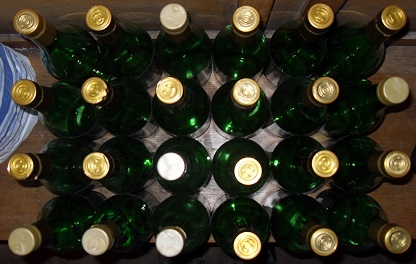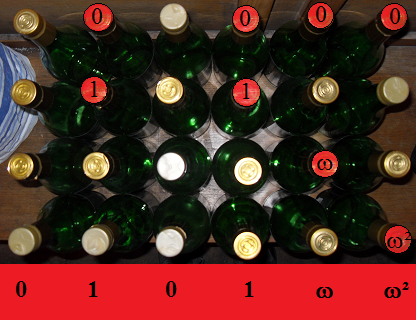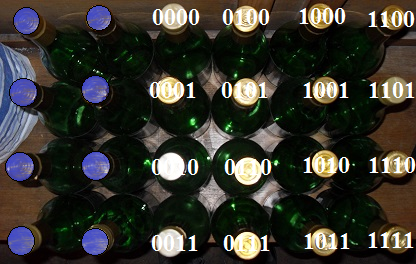# MOG

Since the conception of the European Girls’ Mathematical Olympiad (henceforth abbreviated to EGMO), the British Mathematical Olympiad Committee has run an annual national olympiad specifically for aspiring female mathematicians. The third edition of the Mathematical Olympiad for Girls (MOG) takes place tomorrow, and over 1400 entrants have subscribed.

In its current instantiation, each candidate sits precisely five questions (and these five questions are uniform throughout the country). Unfortunately, this could be prone to collaboration and other slightly mischievous behaviour. At the opposite end of the spectrum, it would be logistically impossible to create different questions for each candidate, and to reliably compare them. In an attempt at compromise, suppose the BMO Committee decreed the following:

There shall be 24 questions in total, and each candidate is given 8 of these. Moreover, no two candidates can have 5 or more questions in common, since that would be conducive to collaboration.

This already imposes an upper limit on the number of girls who can compete. It’s not too difficult to establish an upper bound of 759 (unfortunately too low for this year’s MOG), and it transpires that this upper bound is actually attainable. Producing these 759 special octads (particular 8-subsets of {1, 2, 3, …, 24}) is a non-trivial task.

The special octads are precisely the codewords of Hamming weight 8 in the binary Golay code, which I briefly mentioned in my article about the Leech lattice. There are other ways to generate these octads, without having to endure the overkill of generating the 4096 words of the Golay code and throwing away 3337 of them. One of these is the Miracle Octad Generator of Curtis, which is a useful mathematical tool for exploring the Golay code and Mathieu groups.

Put simply, the Miracle Octad Generator (or MOG) is a 4 × 6 array of things, which may as well be the bottlecaps of these 24 empty bottles of Sandringham Apple Juice:Here is an example of an octad, namely the eight bottlecaps that have been coloured red:For a set of eight points to be a special octad, it needs to exhibit the following properties:

• The numbers of points in each of the six columns must have the same parity;
• The number of points in the top row must also have this parity;
• The column scores must form a hexacodeword.

The first and second conditions can be seen to hold, as the number of points in each column and the top row are all even. The third condition requires further explanation. We assign a score of 0, 1, ω and ω² (in the finite field of four elements) to each point in the first, second, third and fourth rows, respectively, and sum them in columns:A hexacodeword is a word of six symbols over$\mathbb{F}_4$ of the form:

(a, b, c, a + b + c, aω² + bω + c, aω + bω² + c)

In this case, 0101ωω² can be verified to be of this form, so that set of eight points satisfies the third condition and does indeed constitute a special octad.

### The Mathieu group M24

The Mathieu group M24 is the group of permutations of the 24 bottlecaps that map special octads to special octads. A special octad is determined uniquely by a set of 5 points it contains (hence, there are$\binom{24}{5} / \binom{8}{5} = 759$ octads in total), so the Mathieu group is transitive on sets of 5 bottlecaps.

### From M24 to PSL(3,4)…

The MOG is ideally suited to analysing the subgroups of M24, which generally have simple descriptions in terms of the array. These are described in Chapter 11 of Sphere Packings, Lattices and Groups. Of particular interest is the following series of nested subgroups:

• (The stabiliser of the empty set is the entire group, M24);
• The stabiliser of 1 point is the Mathieu group M23;
• The stabiliser of 2 points is the Mathieu group M22;
• The stabiliser of 3 points is M21, directly isomorphic to PSL(3,4).

Indeed, the action of the projective special linear group PSL(3,4) on 21 points is the natural one, with 21 = 4² + 4 + 1 being the number of points on the projective plane over the field of four elements. Hence, this projective plane can be beautifully embedded in the MOG. The three fixed points are coloured blue, labelled I, II and III, and called Romans.The sixteen gold bottlecaps are already in a 4 × 4 grid, which is the natural structure for an affine plane over a field with four elements, where the top-left corner is (0,0) and the top-right corner is (ω²,0) (extrapolate from there). The five green bottlecaps form the line at infinity, with the symbol referring to the value of the gradient y/x of the affine lines that pass through that point.

This can be extended to the projective general linear group PGL(3,4) if we include permutations corresponding to 3 × 3 matrices with determinants ω and ω² as well as those with determinant 1. However, those matrices cyclically permute the three Romans.

In fact, there is another outer automorphism of the projective plane, obtained by the field automorphism of complex conjugation (interchanging ω and its conjugate ω²). These operations double the size of the automorphism group, and correspond to odd permutations of the Romans. This overall group is called PΓL(3,4), or the projective semilinear group, which is a maximal subgroup of M24.

### …and back again

Instead of reducing M24 to obtain PSL(3,4), we can actually use PSL(3,4) to construct the 759 octads in a way that avoids all of that tedious mucking about with the hexacode. Specifically, PSL(3,4) has 360 simple subgroups of order 168 (isomorphic to PSL(2,7)) and 168 simple subgroups of order 360 (isomorphic to the alternating group A6). There are three conjugacy classes of each.

The order-168 subgroups induce seven-point orbits (Fano subplanes), and the order-360 subgroups induce six-point orbits (hyperconics). Now, the 759 octads are:

• The 21 lines of the projective plane, each augmented with all three Romans;
• The 168 hyperconics, each augmented with two Romans depending on the conjugacy class;
• The 360 Fano subplanes, each augmented with one Roman depending on the conjugacy class;
• The 210 symmetric differences of two lines.

The stabiliser of a special octad as a whole (without loss of generality, the standard octad comprising the three Romans together with the line at infinity) acts on the 24 points in two orbits: the octad and its complement, the 16 points of what was previously treated as an affine plane over F4. Instead, we’ll now treat it as an affine 4-space over F2, and the octad group is just the group of affine transformations. The subgroup fixing a point of the affine plane (without loss of generality, the ‘origin’ 0000) is just the general linear group GL(4,2), which is abstractly isomorphic to the alternating group A8. Indeed, it acts faithfully by even permutations of the elements of the octad, so M24 ‘explains’ the exceptional isomorphism between A8 and GL(4,2).Words in the binary Golay code consist of either 0, 8, 12, 16 or 24 points. Those with 8 points are the special octads on which we’ve concentrated so far. Equally interesting, however, are the umbral dodecads of 12 points, which can be constructed by taking the symmetric difference of any two overlapping special octads.

The stabiliser of a dodecad as a whole is the Mathieu group M12. Recall that the octad group together with fixing the origin splits the 24 points into subsets of sizes 1, 8 and 15, and ‘explains’ the exceptional isomorphism between A8 acting on 8 points and GL(4,2) acting on 15 points. Similarly, the dodecad group explains an exceptional isomorphism between M12 and M12 — that is to say, an exceptional outer automorphism. In the same way, M12 splits into two copies of A6 (or it might be S6), explaining the exceptional outer automorphism thereof. There’s actually a miniMOG (known as the kitten, for obvious reasons) to analyse M12, analogous to how the MOG can be used to analyse M24.

This entry was posted in Uncategorized. Bookmark the permalink.

### 0 Responses to MOG

1.Thomas says:

If there are more entrants than the upper bound, can’t they just increase the total number of questions?

•Thomas says:

For which sets (a,b,c) (total number of questions, questions each person gets, and amount they cannot have in common) is the upper bound a!(b-c)!/(b!(a-c)!) obtained?

•apgoucher says:

These are called Steiner systems, and there are many unsolved problems concerning uniqueness. Traditionally, c, b and a are called t, k and n, respectively, and the Steiner system is denoted S(t,k,n).

S(2,3,n) exists if and only if n is congruent to 1 or 3 (modulo 6). S(3,4,n) exists if and only if n is congruent to 2 or 4 (modulo 6). These are known as triple and quadruple systems, respectively.

Some interesting Steiner systems are S(5, 8, 24), S(4, 7, 23), S(3, 6, 22), S(5, 6, 12) and S(4, 5, 11), which have the five Mathieu groups as their automorphism groups (except in the case of S(3, 6, 22), where it’s a double cover of M22).

It is unknown whether there exist any examples with$t \geq 6$, and I believe that the M12 and M24 designs are the only known examples with t = 5.

•Thomas says:

When you mention automorphism groups, is that the group of symmetries of switching the particular sets, or switching the colors? Also, are the possible automorphism groups known?

•apgoucher says:

The automorphism group G is the subgroup of S_n consisting of those permutations which map blocks to blocks. What do you mean by ‘switching the colours’; I don’t recall that colours are ever mentioned in Steiner systems? I don’t think so, not until the Steiner systems are completely classified.

•Thomas says:

Woops, I meant the elements to choose from, not the colours.

•apgoucher says:

Yes, in the case of the Steiner system S(5, 8, 24), the automorphism group M24 is a group of permutations on the set of 24 elements.

•Thomas says:

So, what are the automorphism groups of the systems S(2,3,n) and S(3,4,n)?

2.ernesto says:

There is a relation of this with the 12 coin problem?